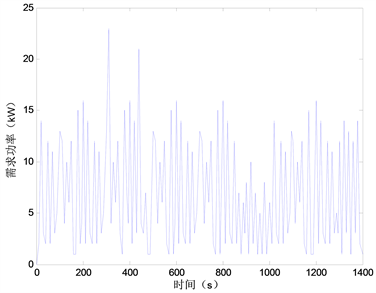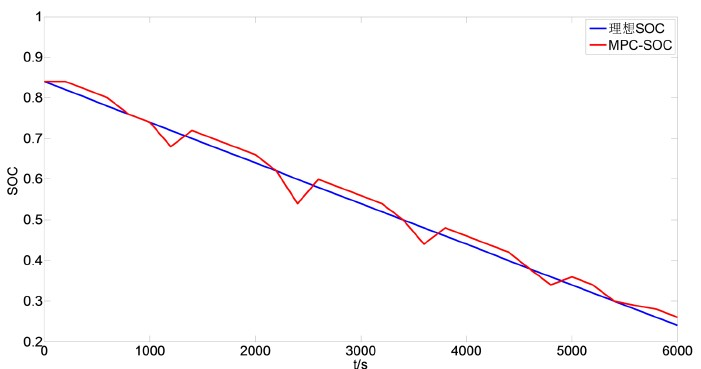#### 期刊菜单

Application of a Dynamic Programming Algorithm Model Predictive Control in Hybrid Electric Vehicle Control Strategy
DOI: 10.12677/DSC.2018.74032, PDF , HTML, XML, 下载: 1,217  浏览: 3,331  科研立项经费支持

Abstract: Good control strategy of hybrid electric vehicles can not only meet the power demand of vehicles, but also effectively save fuel and reduce emissions. In this paper, the construction of model pre-dictive control (MPC) in hybrid electric vehicle (HEV) is proposed. The solving process and the use of reference trajectory are discussed for the application of MPC based on dynamic programming algorithm. The simulation results show that the control method can effectively reduce fuel con-sumption when the torque of engine and motor is reasonably distributed, and the effectiveness of the control strategy is verified.

1. 绪论

2. 基于模型预测控制的混合动力汽车优化控制函数的设计

${J}_{k}=\underset{k}{\overset{k+p}{\sum }}L\left(x\left(t\right),u\left(t\right)\right)=\underset{k}{\overset{k+p}{\sum }}f\left(t\right)$ (1)

3. 基于动态规划在混合电动汽车模型预测控制的应用

3.1. 动态规划在模型预测控制的求解

${J}_{k}^{\ast }\left(SOC\left(k\right)\right)=\underset{u\left(k\right)}{\mathrm{min}}\left[L\left(SOC\left(k\right),u\left(k\right)\right)+{J}_{k+1}^{\ast }\left(SOC\left(k+1\right)\right)\right]$ (2)

3.2. SOC参考轨迹及使用Figure 1. Power demand diagram under cyclic condition

$SO{C}_{r}\left(k\right)=SO{C}_{0}-\frac{k}{s}\left(SO{C}_{0}-SO{C}_{f}\right)$ (3)

$SO{C}_{0}=SO{C}_{i}-0.01$ (4)

${J}_{k}=\underset{k}{\overset{k+p}{\sum }}\left(f\left(t\right)+h\left(SOC\left(t+\text{1}\right)\right)\right)$ (5)

$h\left(SOC\left(t\right)\right)=\left\{\begin{array}{l}0,SOC\left(t\right)\ge SO{C}_{r}\left(t\right)\\ \alpha {\left(SOC\left(t\right)-SO{C}_{r}\left(t\right)\right)}^{2},SOC\left(t\right) (6)

4. 结论Figure 2. SOC curve of storage battery

  彭星. 基于自适应模型预测控制的电动汽车恒速下坡控制研究[D]. 南京农业大学, 2015.  占子奇. 电动汽车再生制动过程的模型预测控制方法研究[D]. 北京工业大学, 2014.  Ripaccioli, G., Bernardini, D., Cairano, S.D., et al. (2010) A Stochastic Model Predictive Control Approach for Series Hybrid Electric Vehicle Power Management. American Control Conference, IEEE, 5844-5849.  Tang, W. and Zhang, Y. (2016) A Model Predictive Control Approach for Low-Complexity Electric Vehicle Charging Scheduling: Optimality and Scalability. IEEE Transactions on Power Systems, PP(99):1-1.  Li, L., Zhang, Y., Yang, C., et al. (2016) Model Predictive Control-Based Efficient Energy Recovery Control Strategy for Regenerative Braking System of Hybrid Electric Bus. Energy Conversion & Management, 111, 299-314. https://doi.org/10.1016/j.enconman.2015.12.077